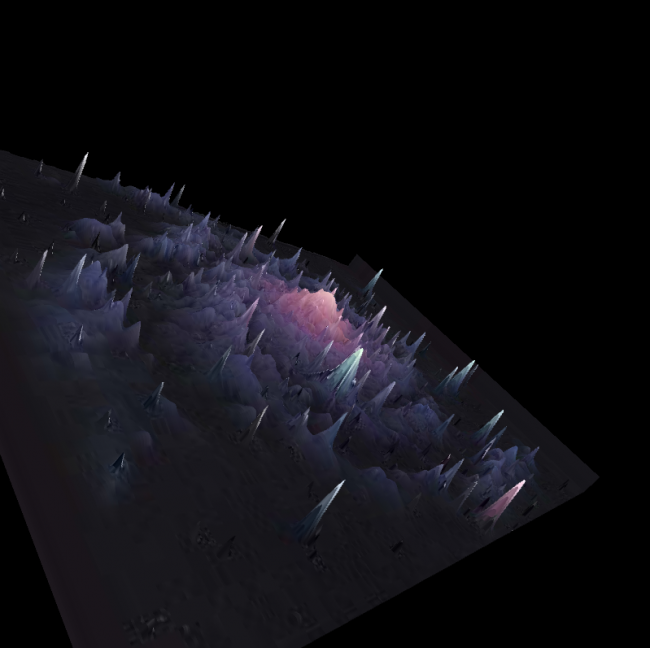#### Howdy, Stranger!

We are about to switch to a new forum software. Until then we have removed the registration on this forum.

# How can I optimize Box drawing ?

edited June 2017

Hi, I've tried to make a program that shows a 2D image in 3D, using the brightness of each pixel. So I draw a box at each x and y, the z is the birghtness. Unfortunately, the only way I've found to see the image is to draw boxes in draw(), but it gets really slow for images bigger than 400*400. I think that there are ways to make that better, so how can I do it ?

Code :

``````import peasy.*;
import peasy.org.apache.commons.math.*;
import peasy.org.apache.commons.math.geometry.*;
PeasyCam camera;
PImage img;

void setup() {
size(800, 800, P3D);
ambientLight(255, 255, 255, 0, 0, 0);
camera = new PeasyCam(this, img.width/2, img.height/2, 0, 1000);
}
void draw() {
background(0);
for (int i=0; i<img.pixels.length; i++) {
color c = img.pixels[i];
float b = map(brightness(c), 0, 255, -100, 100);
fill(c);
noStroke();
int x = i%(img.width);
int y = (i-x)/img.width;
translate(x, y, b);
box(1);
translate(-x, -y, -b);
}
}
``````
Tagged:

• 640,000 boxes...

• The geometry is static so try a PShape. Also, boxes that small probable won't look like boxes so try points instead - no need to draw 6 sides per pixel that way.

But the main problem is the sheer number of boxes you're trying to draw

• I can draw less boxes, but I'll lose resolution. And I can't get the PShape method to work :

``````import peasy.*;
import peasy.org.apache.commons.math.*;
import peasy.org.apache.commons.math.geometry.*;
PeasyCam camera;
PImage img;
PShape particles;

void setup() {
size(800, 800, P3D);
ambientLight(255, 255, 255, 0, 0, 0);
camera = new PeasyCam(this, img.width/2, img.height/2, 0, 1000);
particles = createShape(PShape.GROUP);
for (int i=0; i<img.pixels.length; i++){
PShape part = createShape(BOX,10,10,10);
part.setFill(img.pixels[i]);
part.beginShape();
part.noStroke();
part.endShape();
int x = i%(img.width);
int y = (i-x)/img.width;
translate(x, y, map(brightness(img.pixels[i]), 0, 255, -100, 100));
}
}
void draw() {
background(0);
shape(particles);
println(frameRate);
}
``````
• can't test the code at the moment but that translate at line 24 looks like it needs applying to the part. otherwise where are you specifying the position of the cube within the group?

there is a limit to the amount of things you can put into a shape, depends on your graphics cards. i also find that adding things as children incurs some overhead, as if something iterates through the children one at a time. but you have no choice here, i don't think (unless you want to add your own quads to a single shape, 6 for each cube)

• In your first solution : you could fill an ArrayList of boxes in setup and display the ArrayList

But your 2nd solution without line 30 And some corrections looks great

example for solution 1 with ArrayList holding boxes of type class Box (another topic but same class and similar filling of the class in setup / generate):

``````    import peasy.*;

ArrayList<Box> boxes = new ArrayList();
PeasyCam cam;

// ------------------------------------------------

void setup() {
size(1200, 1000, P3D);
cam = new PeasyCam(this, 0, 0, 0, 200);
println("working");
//boxes=
generate(0, 0, 0,
2,
167);
println("done: "+boxes.size());
}

void draw() {
background(0);
avoidClipping();
lights();
for (int i = 0; i<boxes.size(); i++) {
//for (int i = 0; i<1; i++) {
boxes.get(i).show();
}
}

// ------------------------------------------------------

void generate(float x2, float y2, float z2, int depth, float r) {

PVector pos=new PVector(x2, y2, z2);
int sum ;

for (int x = -1; x < 2; x++) {
for (int y = -1; y < 2; y++) {
for (int z = -1; z < 2; z++) {

sum = abs(x) + abs(y) + abs(z);

if (false) {
if (sum <= 1) {
break;
}
}

float newR = r/3;

if (sum > 1) {
if (depth==0) {
Box b = new Box(pos.x + x*newR,
pos.y + y*newR,
pos.z + z*newR,
newR,
boxes.size());

// if (x!=-1&&y!=0)
// if (random(100)>9)
} else
{
generate(pos.x + x*newR,
pos.y + y*newR,
pos.z + z*newR,
depth-1,
newR);
}
}
}
}
}
// return boxes;
}//func

void avoidClipping() {
// avoid clipping (at camera):
// https : //
// forum.processing.org/two/discussion/4128/quick-q-how-close-is-too-close-why-when-do-3d-objects-disappear
perspective(PI/3.0, (float) width/height, 1, 1000000);
}//func

// ========================================================

class Box {

PVector pos;
int index;

Box(float x, float y, float z,
int index_) {
pos = new PVector(x, y, z);
index = index_;     //index = boxes.size();
}

void show() {
pushMatrix();
translate(pos.x, pos.y, pos.z);

// text
if (false) {
pushMatrix(); // store Matrix
fill(255, 2, 2); //red
textAlign(CENTER, CENTER); // alignment
textMode(SHAPE);           // looks much better
text(index, 0, 0, 0); // this is new
popMatrix(); // restore Matrix
}

// how to draw the box
if (!keyPressed) {
//  noStroke();
stroke(111);
fill(255);//white
fill(255, 0, 0);//white
} else {
noFill();
stroke(255);
}

//box

popMatrix();
}//method
//
}//class
//
``````
• Thank you but with that, I still get low framerate. I've tried with triangles strip and it works great :``````import peasy.*;
import peasy.org.apache.commons.math.*;
import peasy.org.apache.commons.math.geometry.*;
PeasyCam camera;
PImage img;
float[][] z;
int step = 1; // Higher = Less precision but more speed

void setup() {
size(800, 800, P3D);
ambientLight(255, 255, 255, 0, 0, 0);
camera = new PeasyCam(this, img.width/2, img.height/2, 0, 1000);
//z[][] stores values of z for each pixel of the image
z = new float[img.width][img.height];
for (int i=0; i<img.width-1; i++) {
for (int j=0; j<img.height; j++) {
z[i][j] = map(brightness(img.pixels[j*img.width +i]), 0, 255, -25, 25);
}
}
//Smooth the z values
for (int i=1; i<img.width-1; i++) {
for (int j=1; j<img.height-1; j++) {
z[i][j] = (z[i-1][j-1]+z[i-1][j]+z[i-1][j+1]+z[i][j-1]+z[i][j+1]+z[i+1][j-1]+z[i+1][j]+z[i+1][j+1])/8;
}
}
noStroke();
}
void draw() {
background(0);
for (int i=0; i<(img.width-step); i+=step) {
beginShape(TRIANGLE_STRIP);
for (int j=0; j<(img.height-step); j+=step) {
fill(img.pixels[j*img.width +i]);
vertex(i, j, z[i][j]);
vertex(i+step, j, z[i+step][j]);
}
endShape();
}
}
``````
• Not possible to store what draw does in a PShape and display that?